Latest Banking jobs   »

# Reasoning Quizzes Quiz For IRDA AM 2023- 06th May

Directions (1-3): Study the information carefully and answer the questions accordingly.
There are six people J, K, L, M, N, and O having different ages but not necessarily in the same order. K is older to more than two people. Only one person is older than N. K is older than L who is not the youngest. The age of O is more than 32year. The one, who is the second youngest, is 32year old and the one who is second oldest, is 68year old. J is older than N and O.

Q1. Who is the youngest?
(a) K
(b) J
(c) M
(d) Either k or M
(e) None of these

Q2. If the sum of the age of K and L is 74 years than what could be the age of O (in year)?
(a) 43
(b) 40
(c) 44
(d) 30
(e) 31

Q3. If the sum of the age of K and N is 112 years than what will be the age of N (in year)?
(a) 43
(b) 46
(c) 44
(d) 57
(e) None of these

Directions (4-6): Study the information carefully and answer the questions accordingly.
Seven people A, B, C, D, E, F, and G are of different heights but not necessarily in the same order. A is taller than E, but shorter than C. The height of G who is taller than D, is 150cm. The one who is second shortest, is 148cm in height. Two people are shorter than F. Neither A nor E has the height which is a multiple of 3. One of them has a height of 156cm. C is taller than G and F and does not have a height of 170cm. The one who is second tallest, is 170cm in height. D is not the shortest among all.

Q4. Who is the tallest in the group?
(a) B
(b) C
(d) D
(d) A
(e) None of these

Q5. How many people are shorter than G?
(a) Two
(b) One
(c) Four
(d) Three
(e) None of these

Q6. What could be the possible height of E?
(a) 159cm
(b) 155cm
(c) 140cm
(d) 149cm
(e) 150 cm

Directions (7-9): Study the information carefully and answer the questions accordingly.
Five students i.e., M, N, O, K, and L study in class X. All of them have different height. L is shorter than N and taller than M. Not more than one student is taller than K. N is shorter than O. O’s height is 14ft and L’s height is 8ft. O is shorter than K.

Q7. Which of the following is the possible height of K (in feet)?
(a) 15
(b) 12
(c) 8
(d) 10
(e) 9

Q8. How many students are taller than L?
(a) One
(b) Two
(c) Three
(d) Four
(e) None of the above

Q9. Which of the following statement is true?
(a) O is shortest.
(b) N’s height is 16 feet
(c) M is taller than O
(d) L is shorter than O
(e) None of the above

Q10. If it is possible to make a six-letter meaningful word from 2nd, 3rd, 5th, 7th, 9th & 13th letters from the left end of the word “CIRCUMSTANCES”, then which will be the 5th letter of the meaningful word? If more than one such word can be formed then ‘X’ is your answer. If no such word is formed, then ‘Y’ is your answer.
(a) Y
(b) A
(c) I
(d) S
(e) X

Directions (11-12): Study the following information carefully and answer the given questions.
Seven girls N, Z, K, R, J, T and L have different ages. Age of N is 13 years. N is 4 years older than J. R is 2 years older than K. L is 3 years younger than K. L is 5 years old. The difference between ages of L and T is 10 years. Z is 4 years old.

Q11. What is the sum of the ages of J and N?
(a) 14 years
(b) 16 years
(c) 18 years
(d) 20 years
(e) None of these

Q12. Who is the third oldest girl?
(a) Z
(b) R
(c) J
(d) N
(e) None of these

Directions (13-15): Study the following information carefully and answer the given questions.
Eight stones A, B, C, D, E, F, G, and H of different weights are placed in decreasing order of their weights from the left end. C is heavier than only B and F. G is lighter than H which is not the heaviest stone. H and G are heavier than D. D is heavier than E. The second heaviest stone’s weight is 89 kg. There is a difference of 15kg between the weight of D and H.

Q13. How many stones are heavier than B?
(a) One
(b) Two
(c) Three
(d) More than three
(e) Cannot be determined

Q14. What will be the possible weight of G?
(a) 99kg
(b) 90kg
(c) 86kg
(d) 72kg
(e) 70kg

Q15. Which among the following stone is heaviest?
(a) None of these
(b) G
(c) A
(c) B
(c) F

Solutions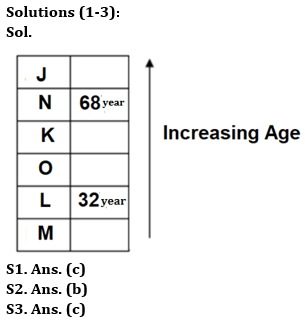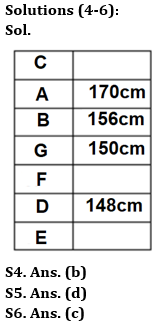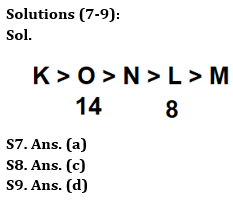S10. Ans. (c)
Sol.2nd, 3rd, 5th, 7th, 9th & 13th letters of the given word are I, R, U, S, A, S. So, the word formed is RUSSIA. Hence, fifth letter of the word is I.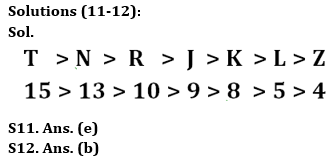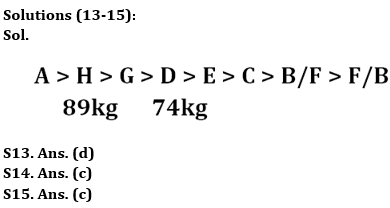## FAQs

### How many sections are there in the IRDAI exam?

There are 4 sections in the IRDAI assistant manager online preliminary exam. They are Reasoning, English Language, General awareness, Quantitative aptitude.

#### Congratulations!Union Budget 2023-24: Free PDF